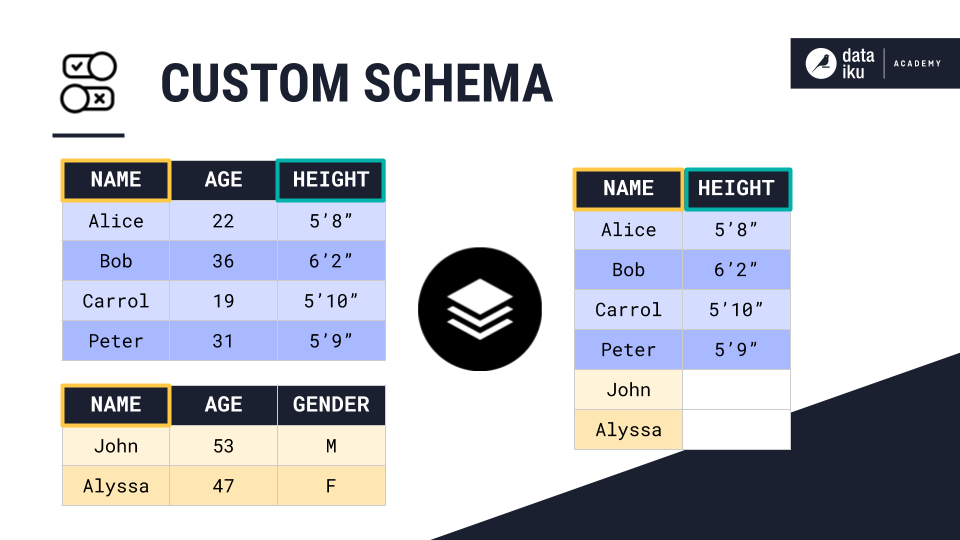# Concept: Stack Recipe¶

The Stack Recipe combines the rows of two or more datasets into a single output dataset. For example, we can use a stack recipe to append the rows from the yellow dataset to those of the blue dataset: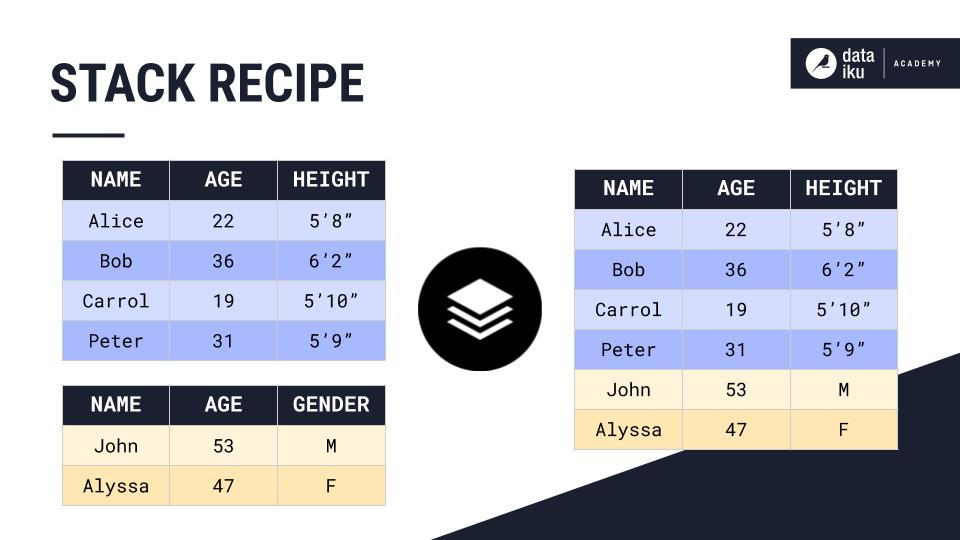The Stack Recipe provides six stacking methods. Each method defines the columns from the input datasets that are included in the output dataset, and the mapping between the columns of the output dataset and the input datasets.

There are six stacking methods in DSS:

• Union of input schemas

• Intersection of input schemas

• Using the schema from one of the input datasets

• Mapping based on the column order of the input datasets

• Manually selecting and remapping columns

• Using a custom defined schema

## Union of Input Schemas¶

The first method is stacking datasets based on the union of input schemas. For example, we can stack the two demographics tables using the Union of input schemas method. As a result, the output dataset contains all of the column names from the input datasets. If there is any missing information, DSS simply creates an empty value.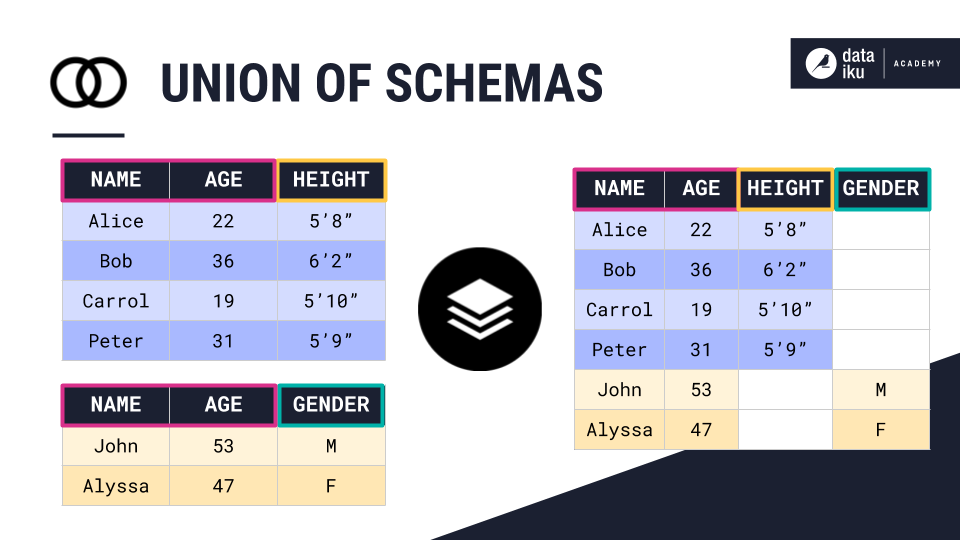## Intersection of Input Datasets¶

The second method is stacking datasets using the intersection of input schemas. For example, we can stack the two demographics tables shown in the example using the Intersection of input schemas method. In this case, the output dataset will only contain column names common to both input datasets.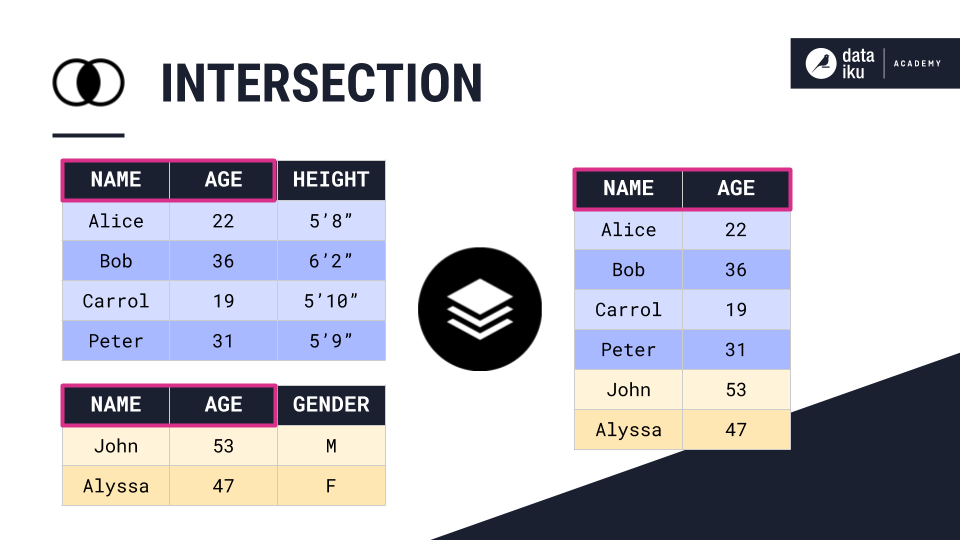## Using the Schema from One of the Input Datasets¶

The third method is stacking datasets using the schema from one of the input datasets. For example, we can stack the two demographics tables using the schema of the blue dataset. In this case, the output dataset only contains column names from the blue input dataset.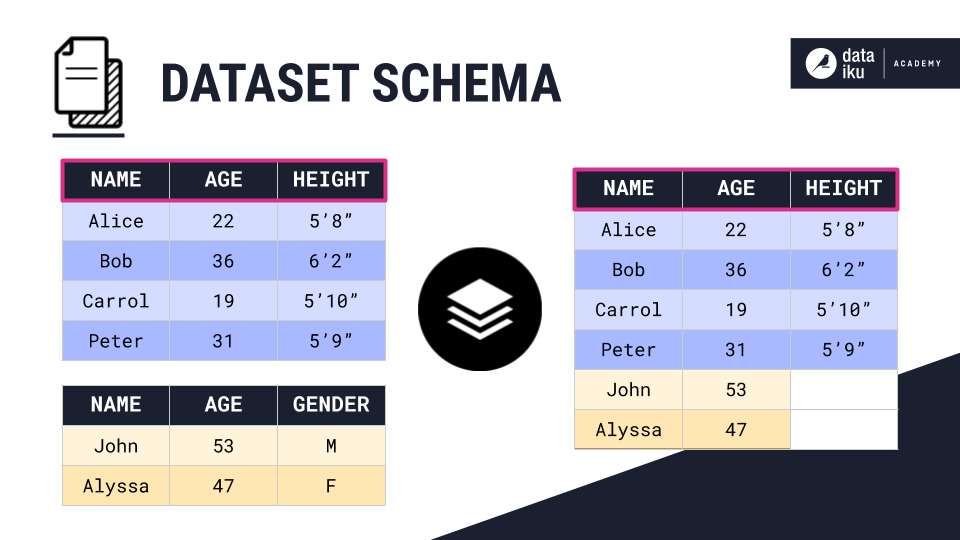Since the yellow input dataset does not have a Height column, DSS pads the output dataset with empty values.

## Mapping Based on the Column Order of the Input Datasets¶

The fourth method is stacking datasets based on column order. For example, we can stack the two demographics tables Using column order. For this stacking method, DSS will match columns based on their ordinal position, ignoring column names. Optionally, you can manually rename column names of the output dataset.

In this example, we have named the third column of the output dataset Wrong, because it is the combination of the Height and Gender columns.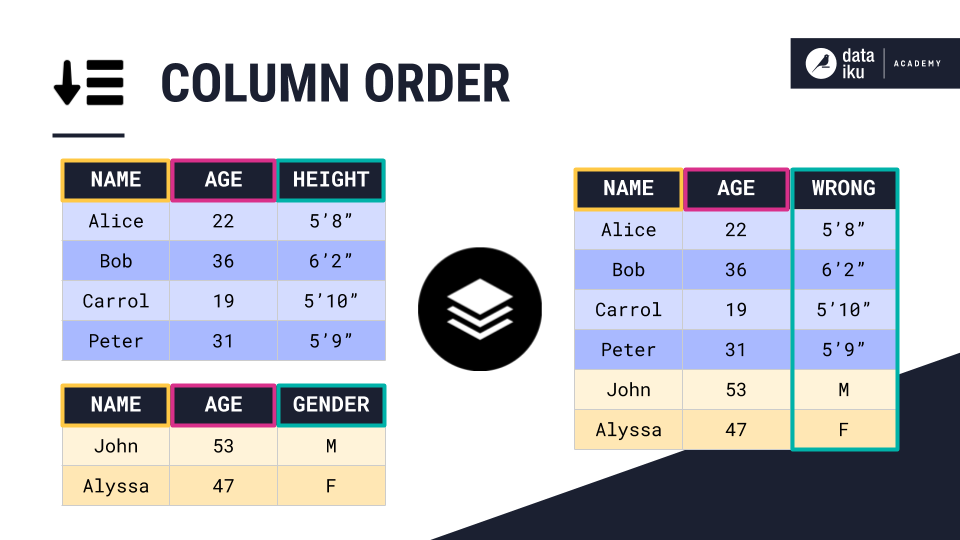## Manually Selecting and Remapping Columns¶

The fifth method is manually selecting and remapping columns. This is the most generic of all available stacking methods. For example, the two demographics tables both contain similar information. However, the order and name of their columns is different.

We can stack them by manually mapping the columns of the output dataset to those of the input datasets. For instance, the Customer column of the output dataset is mapped to the Name column of the blue table, and the Person column of the yellow table.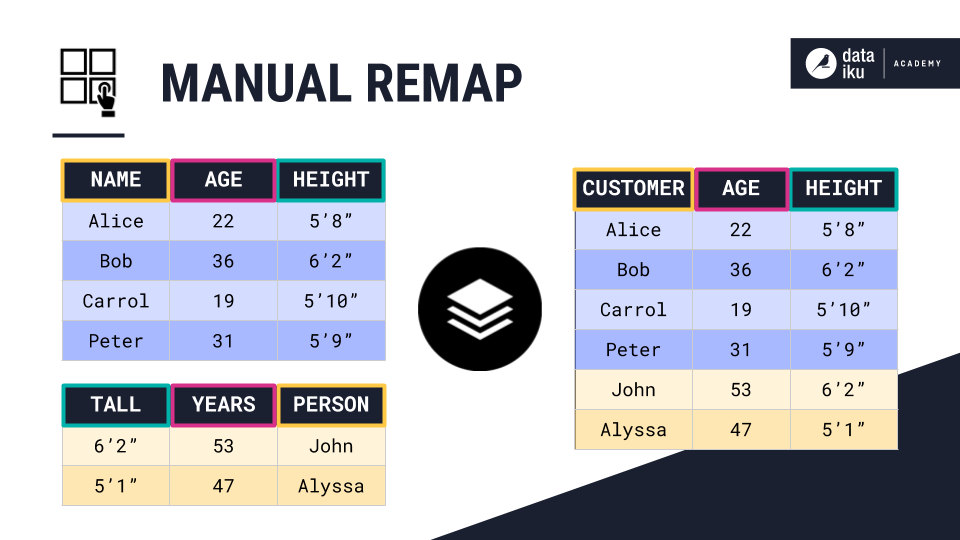## Using a Custom Defined Schema¶

The sixth and final method is stacking datasets based on a custom schema. In this method, we define the schema of the output dataset as a subset of the input schemas. For example, we can join the two demographics tables by defining an output schema consisting of the Name and Height columns.

As a result, DSS will match the columns of the input dataset based on their name, and pad them with empty values if any of the columns are missing.